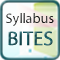# Syllabus bites – proportionality problems

Secondary KLA:
Mathematics
Educational levels:
Year 9, Year 10This is the fourth in a series of Syllabus bites related to direct and indirect proportion. Students use graphs, equations and numerical methods to solve problems involving direct proportion.

## NSW syllabus outcomes

(MA5.2-1WM) selects appropriate notations and conventions to communicate mathematical ideas and solutions

(MA5.2-2WM) interprets mathematical or real-life situations, systematically applying appropriate strategies to solve problems

(MA5.2-5NA) recognises direct and indirect proportion, and solves problems involving direct proportion

## Australian curriculum content descriptions

(ACMNA208) Solve problems involving direct proportion. Explore the relationship between graphs and equations corresponding to simple rate problems

Resource type:
Interactive Resource
ScOT topics:
Direct proportion, Problem solving
File type:
text/html
Language/s:
en-AU
Author:
State of NSW, Department of Education
Publisher:
State of NSW, Department of Education
Date created:
Monday, 12 November 2018